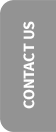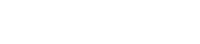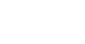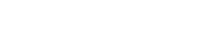# How to use percentages in writing

Posted on January 3, 2020

At this time of year, we start reading and hearing a lot about the year just gone, and how it compared to the year before that. For example, we’ve recently heard that Boxing Day high street shopping was down 8.6% in 2019 compared to 2018, but what do statistics like this mean and how are they calculated?

## Why percentages?

Simply put, a percentage takes something and expresses it as a fraction of 100. For example, if in a group of 10 people there are five with brown hair, you could say that 50% of them have brown hair. It’s very rare that there are exactly 100 of something, but we use 100 because it’s a number we can visualise and base calculations around easily due to our decimal counting system.

Although we think of 100 as a ‘round number’, it’s actually a fairly arbitrary one. Some mathematicians argue we should use the duodecimal system, meaning counting in twelves rather than tens. This would mean new names and symbols would be needed for the numbers 10 and 11, and that the duodecimal number 10 would be the number we know as 12. Thus, in duodecimal counting, ‘100’ would be 12 x 12 rather than 10 x 10, so would equal 144.

The basis for this argument is that 12 is a more mathematically versatile number than 10, because it can be divided by any of 1, 2, 3, 4, 6 and 12 itself. However, the reason we count in tens is probably simply because we have 10 fingers and we use them to help us add up.

Once we’ve settled on 10 as a base, it makes sense to use it to our advantage by basing calculations around it. The metric measuring system does this by, for example, measuring length in kilometres, which are divided into 1,000 metres. A metre is 100 centimetres, and a centimetre is 10 millimetres. This simple, easy-to-calculate system is the one most Europeans grow up with, so it’s understandable why they would find our imperial system – whereby 12 inches make a foot, three feet make a yard, and 1,760 yards make a mile – a bit unwieldy.

So, percentages help simplify ratios. If 2,387 people are asked if they like ice cream, and 2,220 of them say yes, the neatest way to summarise that is to say that 93% of the people asked like ice cream.

## How to calculate percentages of percentages

A common mistake people make is when they try to work out the difference between one percentage and another, and then express that change as a percentage.

For example, let’s imagine that in 2018, 100 people were asked whether they prefer chocolate or strawberry ice cream. The result was a perfect 50-50 split. In 2019, the same question was asked again, but this time, 51 people went for chocolate and 49 chose strawberry.

So from 2018 to 2019, the percentage of people who like chocolate ice cream went up from 50% to 51%. That’s an increase of 1%, isn’t it?

No, it isn’t. Of course, 50 plus 1 is 51, but what you need to remember is that this apparent extra 1% is taken from the original 50%. That means we need to work out 1 out of 50 as a percentage, which is 2%.

So, if something goes up from 50% to 51%, it’s actually gone up by 2%. Confusing, yes, but accurate! If you don’t know where to start with calculations like this, online tools like this one can do the arithmetic for you – all you need to do is put the right numbers in the right boxes.

## How should percentages be written?

Sometimes in news writing, you might see a percentage written in words, like ‘seven percent’ or ‘seven per cent’. However, this starts to look clumsy if you start writing amounts like ‘seventy-four per cent’ or, worse still, ‘seventy-four point six per cent’. For that reason, I prefer to keep percentages consistent and write them as numerals, so ‘7%’ and ‘74.6%’. They are a mathematical concept, after all.

We like percentages here at Engage Web, whether we’re using them in blogs about changes in our clients’ industry, or calculating month-to-month differences in traffic to your website. That’s why we insist on getting them right!

Latest posts by John Murray (see all)